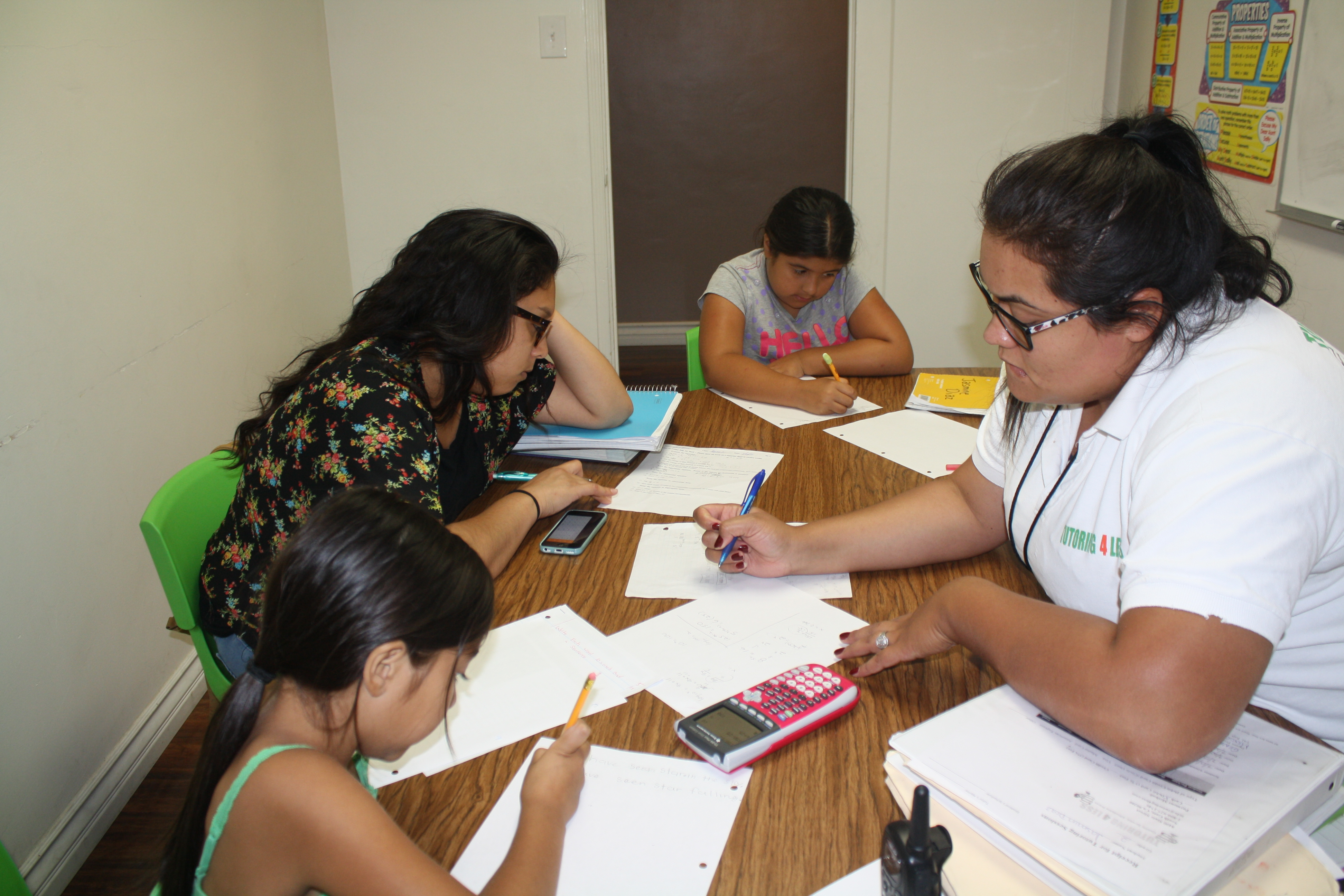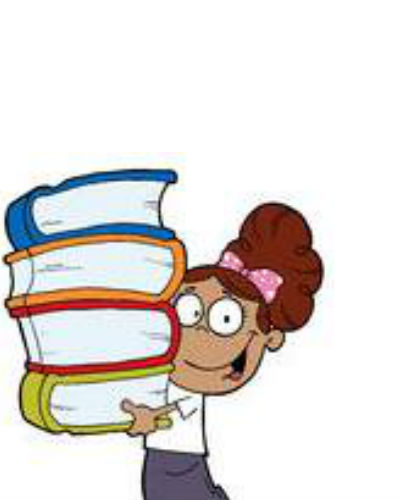#Tutoring 4 Less can provide expert algebra tutoring for young mathematicians who want additional support to get ahead. Our highly experienced math tutors make equations and formulas understandable and interesting. Tutoring 4 Less teaches students specialized techniques for solving algebraic equations and expressing formulas in ways that are much easier to learn than the traditional methods.  By using our customized curriculum that is tailored to suit each student’s needs, we are able to assist students in achieving their academic goals.

## Top Algebra Tutors Near Los Angeles, CA

Tutoring 4 Less teaches LA County students the necessary components of algebra, building on the foundations of mathematical concepts established in elementary school: the rules of operations, relations, functions and their concepts, working with polynomials, solving equations, and algebraic expressions. We also teach students to graph both planar and one-dimensional graphs, determine patterns, and construct tables and matrices of numbers.

Upon completion of our algebra tutoring program, students will be able to demonstrate a higher level of thought processing when approaching problems, and effectively communicate those thoughts using their newly acquired mathematical language.## Online Algebra Tutoring

Our math tutors in Los Angeles for algebra are highly capable of providing quality tuition in either in-person or online tutoring sessions. When looking for an algebra tutor online, it can be hard to find the sweet spot of value for money, experience, and convenience. Tutoring 4 Less provides all of the above. Our online private tutoring can make all the difference for middle school students struggling with algebra.

## Do Algebra Tutors Really Help?

Algebra is the study of symbols (usually represented by letters) and the rules and operations to manipulate these symbols. Algebra helps students predict possible outcomes for specific scenarios and helps them determine the relationships between the things we do and how specific actions and changes can alter them.

Algebra is a powerful branch of mathematics that is useful in everyday life, especially in the worlds of business, engineering, architecture, arts, science, and medicine. Algebra is used in correlation with number theory, geometry, and word analysis to unify all levels and aspects of mathematics. It also continues to be a topic in further mathematical study: high school students use algebra in multiple math classes, including college algebra. Learning algebra isn’t always the same for every student: while the mathematic principles don’t change, different learning styles mean that different teaching approaches may be more successful, especially when it comes to one-on-one tutoring. A math tutor can provide this individual support that makes the difference between complete confusion and total comprehension. The algebra tutors at Tutoring 4 Less have successfully tutored students of many different ability levels and learning styles. If your student needs additional support before teaching algebra is possible, such as pre-algebra, we can provide this.

Upper division algebra differs from basic arithmetic in that it uses abstract symbols, such as letters, which are used to represent unknown or specific values in algebraic expressions. In the famous energy equation, E = mc2, “E” (energy) and “m” (mass) are the varying values, and “c” which represents the speed of light in a vacuum is a constant value at 3 x 108 m/s. By the end of the Tutoring 4 Less algebra program your student will know how to calculate the speed of light!